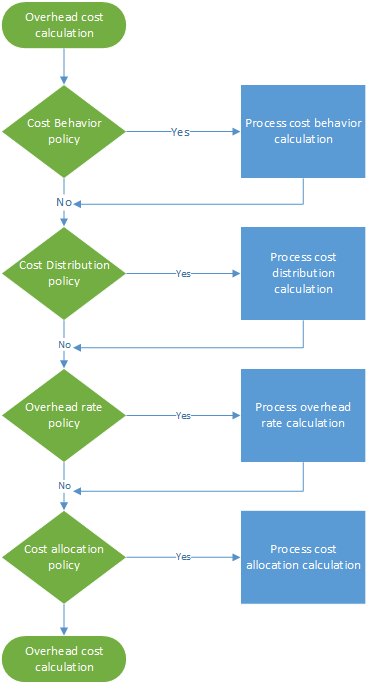# How to Calculate Overhead Costs in Microsoft Dynamics 365 Supply Chain Management

Posted on: February 2, 2023 | By: Guy Logan | Microsoft Dynamics AX/365, Microsoft Dynamics ManufacturingOverhead calculation runs the cost accounting policies in the correct order. You can run overhead calculation multiple times for the same fiscal period if cost accounting policies have been changed or specific errors have been detected. Each run of the overhead calculation is stored and receives a unique version ID that lets you compare the calculations in various versions. The cost entries that the overhead calculation generates receive an accounting date. This accounting date matches the end date of the fiscal period that is used in the calculation. The unique version ID consists of the following elements:

• Version type
• Date and time
• Cost accounting ledger
• Fiscal year
• Fiscal period

Overhead calculation is run independently of the version. Therefore, you can calculate the Budget version before the Actual version. Overhead calculation consists of four steps, as shown in the following illustration. In each step, a journal header is created that has journal entries. This journal header keeps the input data for each calculation step. Policies and rules are applied to each journal line, and cost entries are generated as output. Therefore, you always have full traceability.

## Calculate and allocate the Electricity overhead cost

In Financial accounting, some costs, such as electricity, are registered as a lump sum. Therefore, detailed managerial insight isn’t provided for Cost accounting. In Cost accounting, to provide correct managerial insight across all organizational units and levels, costs must flow through the organizational units. This flow must be based on either an accurate record of the consumption or a fair assessment. In the general ledger, an electricity cost can be posted as shown in the following table.

### Step 1: Process the cost behavior calculation

By default, when cost entries are imported from the source data, they receive the Unclassified cost behavior classification in Cost accounting. By applying cost behavior policy rules, you can reclassify cost entries as either Fixed cost or Variable cost.

### Step 2: Process the cost distribution calculation

Cost distribution is used to redistribute cost from one cost object to one or more other cost objects by applying a relevant allocation base. Cost distribution and cost allocation differ in that cost distribution always occurs at the level of the primary cost element of the original cost.

### Step 3: Process the overhead rate calculation

The overhead rate is used to charge one or more specific cost objects. The charge is based on a predetermined cost rate and the magnitude from the assigned allocation base.

### Step 4: Process the cost allocation calculation

Allocation is used to allocate the balance of a cost object to other cost objects by applying an allocation base. Finance supports the reciprocal allocation method. In the reciprocal allocation method, the mutual services that auxiliary cost objects exchange are fully recognized. The system automatically determines the correct order to perform the allocations in. The balance of a cost object is allocated by a single allocation base. Allocations across cost objects dimensions and their respective members are supported. The allocation order is controlled by the cost control unit.

### Next Steps

If you are interested in learning more about how to calculate overhead costs with Microsoft Dynamics 365 for Supply Chain Management, contact us here to find out how we can help you grow your business. You can also email us at info@loganconsulting.com or call (312) 345-8817.

2020 Nucleus Research Report on ERP Technology